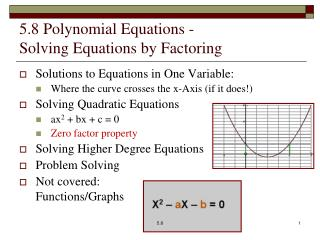DownloadDownload Presentation5.8 Polynomial Equations - Solving Equations by Factoring

# 5.8 Polynomial Equations - Solving Equations by Factoring

Download Presentation## 5.8 Polynomial Equations - Solving Equations by Factoring

- - - - - - - - - - - - - - - - - - - - - - - - - - - E N D - - - - - - - - - - - - - - - - - - - - - - - - - - -
##### Presentation Transcript

1. 5.8 Polynomial Equations - Solving Equations by Factoring • Solutions to Equations in One Variable: • Where the curve crosses the x-Axis (if it does!) • Solving Quadratic Equations • ax2 + bx + c = 0 • Zero factor property • Solving Higher Degree Equations • Problem Solving • Not covered:Functions/Graphs 5.8

2. Curves and x-Intercepts • y=x+3 y=x2 y=x3y=|x| 5.8

3. Definition Zero Factor Property 5.8

4. Examples: 0, 1, or 2 solutions Unfactorable quadratics are prime and have no rational number solutions. Rewrite Factor Set Each = 0 Solve Each Check 5.8

5. Practice: 0, 1, or 2 solutions Unfactorable quadratics are prime and have no rational number solutions. Rewrite Factor Set Each = 0 Solve Each Check 5.8

6. Higher Degree Polynomial Equations • Write in descending powers of the variable = 0 • Make leading coefficient positive (multiply by -1) • Factor • Solve each factor = 0 • May have 0 to n (the degree) solutions • Check all solutions in original equation 5.8

7. Examples, 0 to n solutions Unfactorable quadratics are prime and have no rational number solutions. Rewrite Factor Set Each = 0 Solve Each Check 5.8

8. Practice, 0 to n solutions Unfactorable quadratics are prime and have no rational number solutions. Rewrite Factor Set Each = 0 Solve Each Check 5.8

9. Integers • Consecutive Integers: n, n+1, n+2, n+3, … • Consecutive Even or Odd Integers:n, n+2, n+4, n+6, … • Find 3 consecutive odd integers where the product of the first 2 is 68 more than the 3rd integer. 5.8

10. Borders 5.8

11. Gravity SLINGSHOT A slingshot can provide an initial velocity of 128 feet per second. At what times will a stone, shot vertically upward, be 192 feet above the ground? 5.8

12. What Next? • 6.1 Rational Expressions • Look for patterns … 5.8